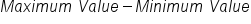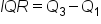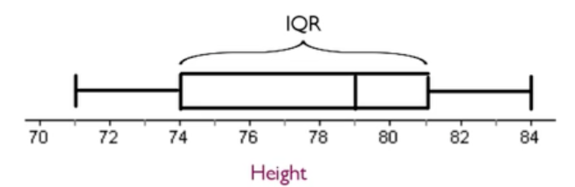+
Range and Interquartile Range (IQR)
Central Tendency, Variation, and Distributions
0
of 90 possible points
Common Core: S.ID.2

# Range and Interquartile Range (IQR)

Author: Sophia Tutorial
##### Description:

Identify the percentage of data in each quartile and interquartile range.

(more)

Sophia’s self-paced online courses are a great way to save time and money as you earn credits eligible for transfer to many different colleges and universities.*

No credit card required

46 Sophia partners guarantee credit transfer.

299 Institutions have accepted or given pre-approval for credit transfer.

* The American Council on Education's College Credit Recommendation Service (ACE Credit®) has evaluated and recommended college credit for 33 of Sophia’s online courses. Many different colleges and universities consider ACE CREDIT recommendations in determining the applicability to their course and degree programs.

Tutorial
what's covered
This tutorial will explain the range and interquartile range (IQR). Our discussion breaks down as follows:

1. Range
2. Interquartile Range

## 1. Range

One measure of variation is range. The range is one of the simplest ways to calculate variation. It is calculated by simply subtracting the minimum value from the maximum value.

formula
RangeEXAMPLE

This chart shows the height of the Chicago Bulls basketball team for a particular year:
Height of Chicago Bulls Players
Omer Asik 84
Carlos Boozer 81
Ronnie Brewer 79
Jimmy Butler 79
Luol Deng 81
Taj Gibson 81
Richard Hamilton 79
Mike James 74
Kyle Korver 79
John Lucas III 71
Joakim Noah 83
Derrick Rose 75
Brian Scalabrine 81
Marquis Teague 74
C.J. Watson 74

It's easy to see from the list that the minimum value is 71 and the maximum value is 84. The range is actually the easiest measure of spread or variation to find: take the maximum value (in this case, the tallest person) and subtract the minimum value (in this case, the shortest person).

Therefore, 84 minus 71 equals 13 inches. This means that every individual on the team falls within a 13-inch range.

term to know

Range
The difference between the largest and smallest number in a data set.

## 2. Interquartile Range

Range and interquartile range are similar ideas in that they're both measured by subtraction, so they're not particularly difficult to calculate. While they are calculated similarly, they do measure different measures of variation.

The interquartile range, also abbreviated IQR, is another measure of spread, but it's median based. To review, the median is the middle number of an ordered data set.

The IQR represents the range in which the middle 50% of the data points lie. Finding the interquartile range takes a few steps.

step by step

Step 1: First, order the data set from smallest to largest and then work your way in towards the center until you find the median.

## 71, 74, 74, 74, 75, 79, 79, 79, 79, 81, 81, 81, 81, 83, 84

The median, in this case, is 79.

Step 2: Once you identify the two data sets on either side of the median--a small half and a high half--you can find the middle number of each of those halves. These three numbers together are called the quartiles.

• The first quartile, or Q1, is where 1/4 of the data falls at or below this point.

• The median, which can also be called the second quartile (Q2), is where half the data falls at or below this point.
• The third quartile, or Q3, is where 3/4 of the data falls at or below this point.

With this data set:

## 71, 74, 74, 74, 75, 79, 79, 79, 79, 81, 81, 81, 81, 83, 84,

• Q1 = 74
• Q2 (median) = 79
• Q3 = 81

Step 3: Finally, to calculate the interquartile range, find the difference between the third and first quartiles.

formula
Interquartile RangeGoing back to our example, the IQR is calculated as Q3 minus Q1, or 81 minus 74, which equals 7 inches. This means that the middle half of the data set falls within a 7-inch range, whereas the entire data set falls within a 13-inch range.

Visually, the IQR is the box on a box plot, shown below:The range gives the entire spread of the data set lowest to highest whereas the IQR gives the range of the middle 50%.

hint
The advantage of using IQR over the range is if there are outliers, which would disproportionately affect the range, the IQR will not be affected by them.

term to know

Interquartile Range
The difference between the third and first quartiles. It represents the range in which the middle 50% of the data points lie.

summary
The range is not the most useful measure of variation, but it is the easiest to calculate. The interquartile range is more useful and measures the range of the middle 50%, the most typical middle 50% of the data. It's a useful measure of spread for distributions with outliers or skewed distribution. In fact, you should use IQR as your measure of variation when those factors are a concern. Because the IQR is based on finding the median, it should only be used as the measure of spread when the median is the measure of center. You should not, for instance, use the mean as the measure of center and then report IQR as the measure of spread.

Good luck!

Source: Adapted from Sophia tutorial by Jonathan Osters.

Terms to Know
Interquartile Range

The difference between the third and first quartiles. It represents the range in which the middle 50% of the data points lie.

Range

The difference between the largest and smallest number in a data set.

Formulas to Know
Interquartile RangeRangeRating